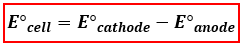# Problem: Use the standard half-cell potentials listed below to calculate the standard cell potential, E°cell, for the following reaction occurring in an electrochemical cell at 25°C. (The equation is balanced.)3 Br2(l) + 2 Fe(s) → 6Br1-(aq) + 2Fe2+(aq)Br2(l) + 2 e- → 2 Br1-(aq)                 E° = +1.09 VFe2+(aq) + 2 e- → Fe(s)                   E° = -0.45 VA) +0.64VB) -0.64 VC) -1.54 VD) +1.54 VE) the standard cell potential is 0.0v

###### FREE Expert Solution
99% (134 ratings)
###### FREE Expert Solution

We’re being asked to determine the standard cell potential (E°cellof the reaction occurring in an electrochemical cell. To calculate for the standard cell potential, use the following equation:When a balanced reaction is given, identify the anode and the cathode half-reactions by determining the changes in oxidation states of each species based on the given reaction:

Recall:

99% (134 ratings)###### Problem Details

Use the standard half-cell potentials listed below to calculate the standard cell potential, E°cell, for the following reaction occurring in an electrochemical cell at 25°C. (The equation is balanced.)
3 Br2(l) + 2 Fe(s) → 6Br1-(aq) + 2Fe2+(aq)
Br2(l) + 2 e- → 2 Br1-(aq)                 E° = +1.09 V
Fe2+(aq) + 2 e- → Fe(s)                   E° = -0.45 V

A) +0.64V
B) -0.64 V
C) -1.54 V
D) +1.54 V
E) the standard cell potential is 0.0v

Frequently Asked Questions

What scientific concept do you need to know in order to solve this problem?

Our tutors have indicated that to solve this problem you will need to apply the Cell Potential concept. If you need more Cell Potential practice, you can also practice Cell Potential practice problems.

What is the difficulty of this problem?

Our tutors rated the difficulty ofUse the standard half-cell potentials listed below to calcul...as medium difficulty.

How long does this problem take to solve?

Our expert Chemistry tutor, Jules took 3 minutes and 54 seconds to solve this problem. You can follow their steps in the video explanation above.

What professor is this problem relevant for?

Based on our data, we think this problem is relevant for Professor Marzal's class at UCF.